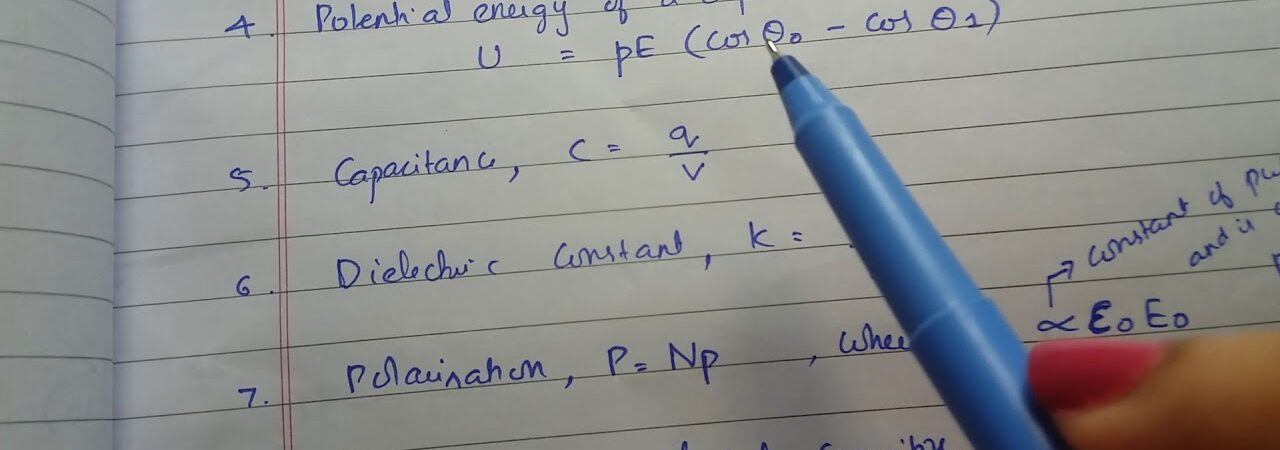# Can I start current electricity class 12 without studying electric potential and capacitance?

To answer the above question we need to first understand how the three topics are related to each other. It is basically important to know all the three topics in depth because they are inter related or inter connected to each other. Let us go through each of them one by one.

1. Current electricity
2. Electric potential
3. Capacitance

Current Electricity:  It is a stream of particles which are charged and some of the examples are ions and electrons. They go through a space which is electrically charged and it is usually the space or a conductor which allows the electricity to pass through. How do we measure it – it is the rate of flow of charge which is electric and it passes through the surface or into a controlled space of volume. The particles which move around in that space is called the carriers of electricity or charge carriers. The factor depends on the conductor and it may be one of many types of particles. Here in the electric circuits the charge carriers are the electrons that move around through a wire.

In the case of semi conductors here we have many holes or electrons and thus in an electrolyte the ions are the ones which carry the charge.

When we talk of electric current the SI unit is the Ampere, also called amp and thus the flow of electric charge at one coulomb per second. We use the symbol A as the base unit. We usually measure the electric current in a device known as the ammeter. Electric currents are known to cause magnetic fields which are widely used by motors and inductors and other devices.

Along with it let us check the alternating and direct currents: The main difference between alternating and direct currents is that in the first instance the electric charge movement is always moving and it always reverses direction. It is that form of electric power that goes for commercial business use and also households. The usual wave form for a AC power circuit is that it goes in a sine wave although there are instances that there can be square or triangular waves. The best example that can be given for the AC current is the radio signals and audio signals on electric wires. IF we go to say what is the application goal is to information recovery for the AC signal.

Direct Currents or DC is the system where the movement of electric charge is only for one particular direction which can also be said to be flow in one direction. Direct current is produced by some sources like the battery, solar cells etc. The alternating currents are also converted to direct current sometimes by using a rectifier. When Direct current flows through a conductor it can also flow via other mediums like insulators and others. Direct currents were used to be known as galvanic currents.

CAPACITANCE:

it is the ratio of electric current which is stored in a conductor to the electric potential. When we talk of capacitance then we need to know they are of two types – self capacitance and mutual capacitance. The SI unit is known as Farad. IF we talk of one Farad capacitor which when charged with one coulomb of electric charge has the potential difference of one volt. When any object is said to conduct electrically charge then it can be said to have capacitance. Here the electric potential difference is measured. When something is said to have more self capacitance then it will hold more electric charge at any potential difference as compared to any one with low capacitance.

Can you answer this question:The variation of potential with distance R from the fixed point. The electric field at R = 5 m is.?

ELECTRIC POTENTIAL: It is also known as the electric field potential or potential drop and it is the amount of energy which is required for one unit of electric charge from a point to another point on the electric field.It is the energy per unit of electric charge for any given charge that is small and thus the disturbance caused by it can be termed negligible. Thus an electric potential is the zero number of units for any reference point. In the context of electrostatics, the electrostatic field is the vector product for a electrostatic potential and this is a scalar quantity.

Thus we see all the above three definitions for electric current and capacitance and also electric potential that these are interrelated and thus one cannot understand one without the other. However since the basis of the content is electric current thus it is important to understand the concepts first for Electric current.